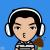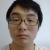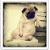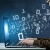# 查看 MySQL 数据库中每个表占用的空间大小

TABLE_SCHEMA : 数据库名
TABLE_NAME：表名
ENGINE：所使用的存储引擎
TABLES_ROWS：记录数
DATA_LENGTH：数据大小
INDEX_LENGTH：索引大小

SQL:

SELECT TABLE_NAME,DATA_LENGTH+INDEX_LENGTH,TABLE_ROWS FROM TABLES WHERE TABLE_SCHEMA='数据库名' AND TABLE_NAME='表名'

31、进去指定schema 数据库（存放了其他的数据库的信息）
mysql> use information_schema;
Database changed
2、查询所有数据的大小
mysql> select concat(round(sum(DATA_LENGTH/1024/1024), 2), 'MB')
-> as data from TABLES;
+-----------+
| data      |
+-----------+
| 6674.48MB |
+-----------+
1 row in set (16.81 sec)
3、查看指定数据库实例的大小，比如说数据库 forexpert
mysql> select concat(round(sum(DATA_LENGTH/1024/1024), 2), 'MB')
-> as data from TABLES where table_schema='forexpert';
+-----------+
| data      |
+-----------+
| 6542.30MB |
+-----------+
1 row in set (7.47 sec)
4、查看指定数据库的表的大小，比如说数据库 forexpert 中的 member 表
mysql> select concat(round(sum(DATA_LENGTH/1024/1024),2),'MB') as data
-> from TABLES where table_schema='forexpert'
-> and table_name='member';
+--------+
| data   |
+--------+
| 2.52MB |
+--------+
1 row in set (1.88 sec)

0#### 引用来自“铂金小熊”的答案

1、进去指定schema 数据库（存放了其他的数据库的信息）
mysql> use information_schema;
Database changed
2、查询所有数据的大小
mysql> select concat(round(sum(DATA_LENGTH/1024/1024), 2), 'MB')
-> as data from TABLES;
+-----------+
| data      |
+-----------+
| 6674.48MB |
+-----------+
1 row in set (16.81 sec)
3、查看指定数据库实例的大小，比如说数据库 forexpert
mysql> select concat(round(sum(DATA_LENGTH/1024/1024), 2), 'MB')
-> as data from TABLES where table_schema='forexpert';
+-----------+
| data      |
+-----------+
| 6542.30MB |
+-----------+
1 row in set (7.47 sec)
4、查看指定数据库的表的大小，比如说数据库 forexpert 中的 member 表
mysql> select concat(round(sum(DATA_LENGTH/1024/1024),2),'MB') as data
-> from TABLES where table_schema='forexpert'
-> and table_name='member';
+--------+
| data   |
+--------+
| 2.52MB |
+--------+
1 row in set (1.88 sec)

00SELECT TABLE_NAME,DATA_LENGTH+INDEX_LENGTH,TABLE_ROWS,concat(round((DATA_LENGTH+INDEX_LENGTH)/1024/1024,2), 'MB') as data FROM TABLES WHERE TABLE_SCHEMA='dbname';